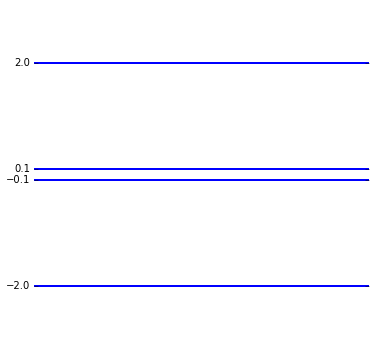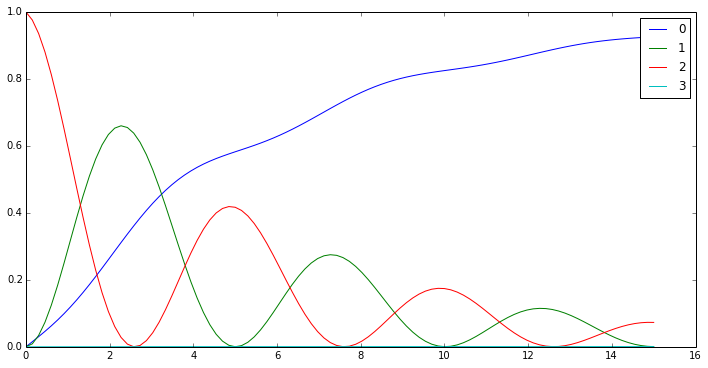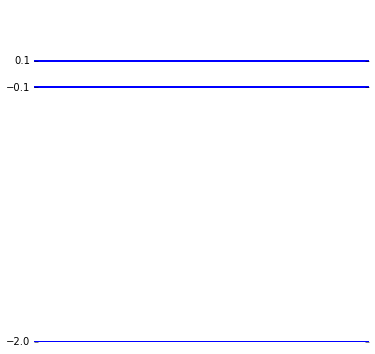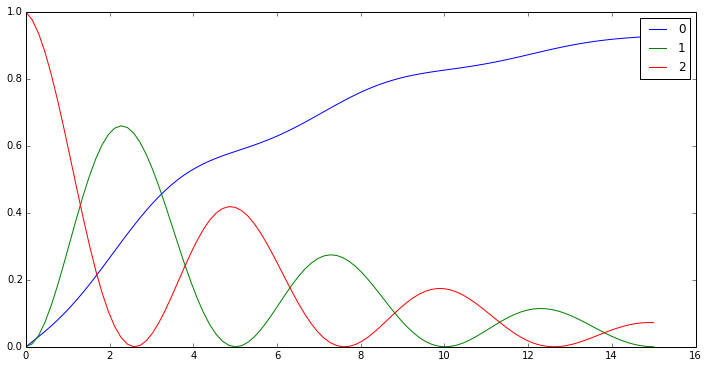### QuTiP development notebook for testing extracting and eliminating states from a quantum object¶

Copyright (C) 2011 and later, Paul D. Nation & Robert J. Johansson

In :
%pylab inline

Populating the interactive namespace from numpy and matplotlib

In :
from qutip import *

In :
def visualize(result):
fig, ax = subplots(figsize=(12,6))
for idx, e in enumerate(result.expect):
ax.plot(result.times, e, label="%d" % idx)
ax.legend()


Consider two coupled qubits:

In :
H0 = -2*pi*(tensor(sigmaz(), identity(2)) + tensor(identity(2), sigmaz()))
Hint = 2*pi*0.1 * tensor(sigmax(), sigmax())

H = H0 + Hint

In :
H

Out:
$\text{Quantum object: dims = [[2, 2], [2, 2]], shape = [4, 4], type = oper, isHerm = True}\\[1em]\begin{pmatrix}-12.566 & 0.0 & 0.0 & 0.628\\0.0 & 0.0 & 0.628 & 0.0\\0.0 & 0.628 & 0.0 & 0.0\\0.628 & 0.0 & 0.0 & 12.566\\\end{pmatrix}$
In :
energy_level_diagram([H], figsize=(6,6), show_ylabels=True);If the highest energy state is not initially occupied, and if we neglect thermal excitations, it will never be occupied, and therefore not really necessary to include in a calculation of for example the dynamics of the three lowest states.

In :
psi_gg = tensor(basis(2, 0), basis(2, 0))
psi_eg = tensor(basis(2, 1), basis(2, 0))
psi_ge = tensor(basis(2, 0), basis(2, 1))
psi_ee = tensor(basis(2, 1), basis(2, 1))

c_op1 = tensor(destroy(2), identity(2))
c_op2 = tensor(identity(2), destroy(2))

In :
psi0 = psi_eg

In :
tlist = linspace(0, 15, 100)
result = mesolve(H, psi0, tlist,
[sqrt(0.1) * c_op1, sqrt(0.25) * c_op2],
[ket2dm(psi_gg), ket2dm(psi_ge), ket2dm(psi_eg), ket2dm(psi_ee)])

In :
visualize(result)Note that the fourth state is not included in the dynamics at all. So...

### Assume we want to create a new Qobj instance using the three lowest states:¶

In :
# We are only interested in these states
keep_states = [0, 1, 2]

# or equivalently, not interested in these states
remove_states = 

In :
H_sub = H.eliminate_states(remove_states)

H_sub

Out:
$\text{Quantum object: dims = [, ], shape = [3, 3], type = oper, isHerm = True}\\[1em]\begin{pmatrix}-12.566 & 0.0 & 0.0\\0.0 & 0.0 & 0.628\\0.0 & 0.628 & 0.0\\\end{pmatrix}$
In :
H_sub = H.extract_states(keep_states)

H_sub

Out:
$\text{Quantum object: dims = [, ], shape = [3, 3], type = oper, isHerm = True}\\[1em]\begin{pmatrix}-12.566 & 0.0 & 0.0\\0.0 & 0.0 & 0.628\\0.0 & 0.628 & 0.0\\\end{pmatrix}$
In :
energy_level_diagram([H_sub], figsize=(6,6), show_ylabels=True);In :
psi0_sub = psi0.extract_states(keep_states, normalize=True)

psi0_sub

Out:
$\text{Quantum object: dims = [, ], shape = [3, 1], type = ket}\\[1em]\begin{pmatrix}0.0\\0.0\\1.0\\\end{pmatrix}$
In :
c_op1_sub = c_op1.extract_states(keep_states)
c_op2_sub = c_op2.extract_states(keep_states)

In :
psi_gg_sub = psi_gg.extract_states(keep_states)
psi_eg_sub = psi_eg.extract_states(keep_states)
psi_ge_sub = psi_ge.extract_states(keep_states)

In :
tlist = linspace(0, 15, 100)
result = mesolve(H_sub, psi0_sub, tlist,
[sqrt(0.1) * c_op1_sub, sqrt(0.25) * c_op2_sub],
[ket2dm(psi_gg_sub), ket2dm(psi_ge_sub), ket2dm(psi_eg_sub)])

In :
visualize(result)Voila! Same results with fewer quantum states in the calculation, and therefore potentially more efficient.

### Software versions¶

In :
from qutip.ipynbtools import version_table
version_table()

Out:
SoftwareVersion
Cython0.17.4
SciPy0.11.0
QuTiP2.3.0.dev-fcfbaeb
Python2.7.4 (default, Apr 19 2013, 18:28:01) [GCC 4.7.3]
IPython2.0.0-dev
OSposix [linux2]
Numpy1.7.1
matplotlib1.4.x
Sat Aug 24 21:29:47 2013 JST
In [ ]: